# 什么是算法

eg:解决两数相加的问题

// 计算a和b的和
public static int plue(int a, int b){
return a + b;
}



eg:解决 n个数字的和 的问题

// 计算1+2+3+...+n
public static int sum(int n){
int result = 0;
for(int i = 1; i <= n; i++){
result += i;
}
return result;
}



# 如何评判一个算法的好坏

• 比较不同算法对同一组输入的执行处理时间
• 这种方案也叫做：事后统计法

• 执行时间严重依赖硬件以及运行时各种不确定的环境因素
• 必须编写相应的测算代码
• 测试数据的选择比较难保证公正性

• 正确性、可读性、健壮性（对不合理输入的反应能力和处理能力）
• 时间复杂度（time complexity） 估算程序指令的执行次数（执行时间）
• 空间复杂度（space complexity）估算所需占用的存储空间

package cn.liuawen;

public class demo {
// 计算 1+2+3+...+n 的和
public static int sum1(int n) {
int result = 0;
for (int i = 1; i <= n; i++) {
result += i;
}
return result;
}

// 计算 1+2+3+...+n 的和
public static int sum2(int n) {
return (1 + n) * n / 2;
}

public static void main(String[] args) {
System.out.println("sum1(10):"+sum1(10));
System.out.println("sum2(10):"+sum2(10));

}
}



# 大O表示法 Big O

• 9 >> O(1)
• 2n + 3 >> O(n)
• n2 + 2n + 6 >> O(n2)
• 4n3 + 3n2 + 22n + 100 >> O(n3)
• 写法上，n3 等价于 n^3’

# 对数阶的细节

• log29 ∗ log9n = og2n# 常见的复杂度

logn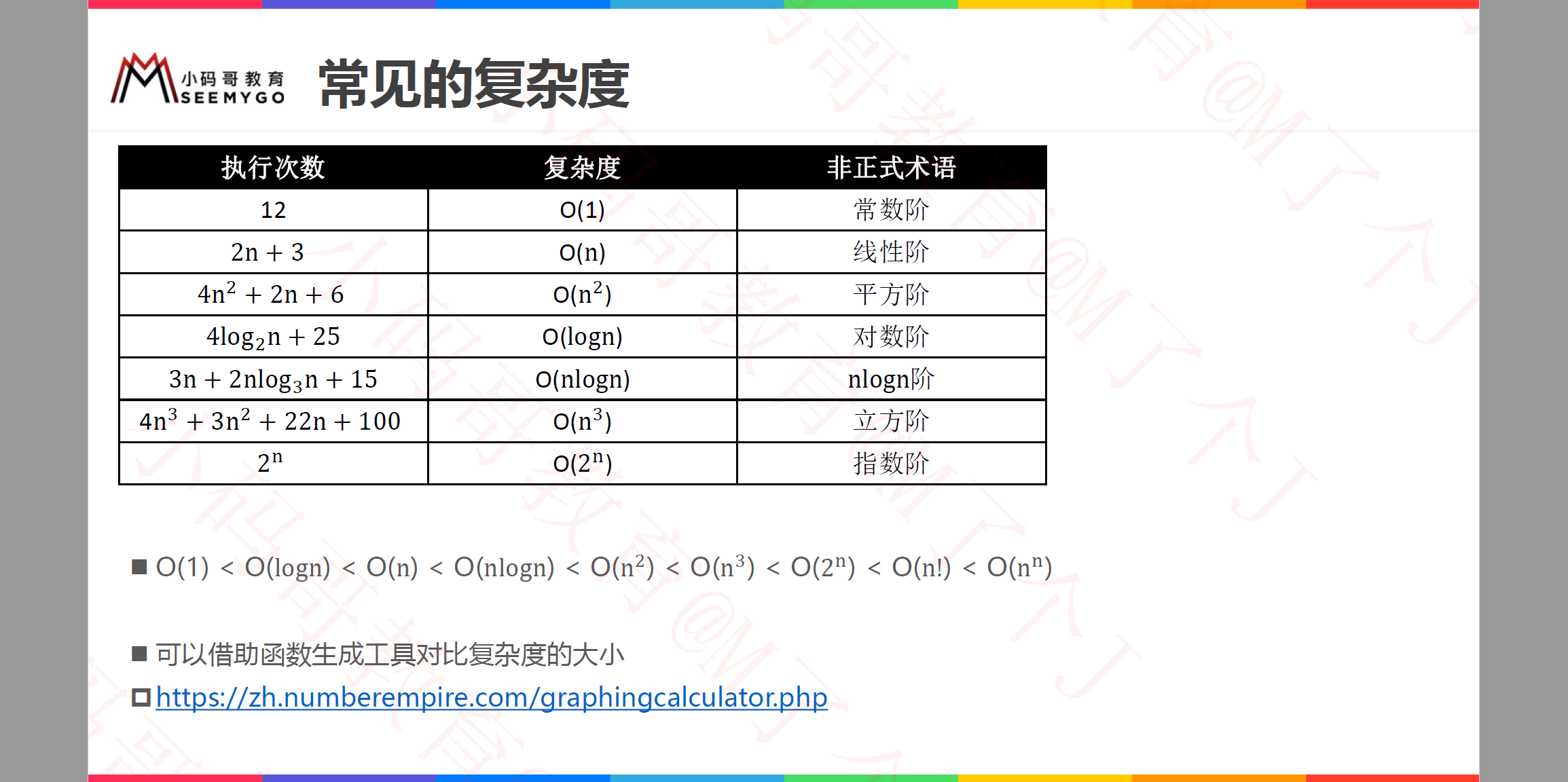https://zh.numberempire.com/graphingcalculator.php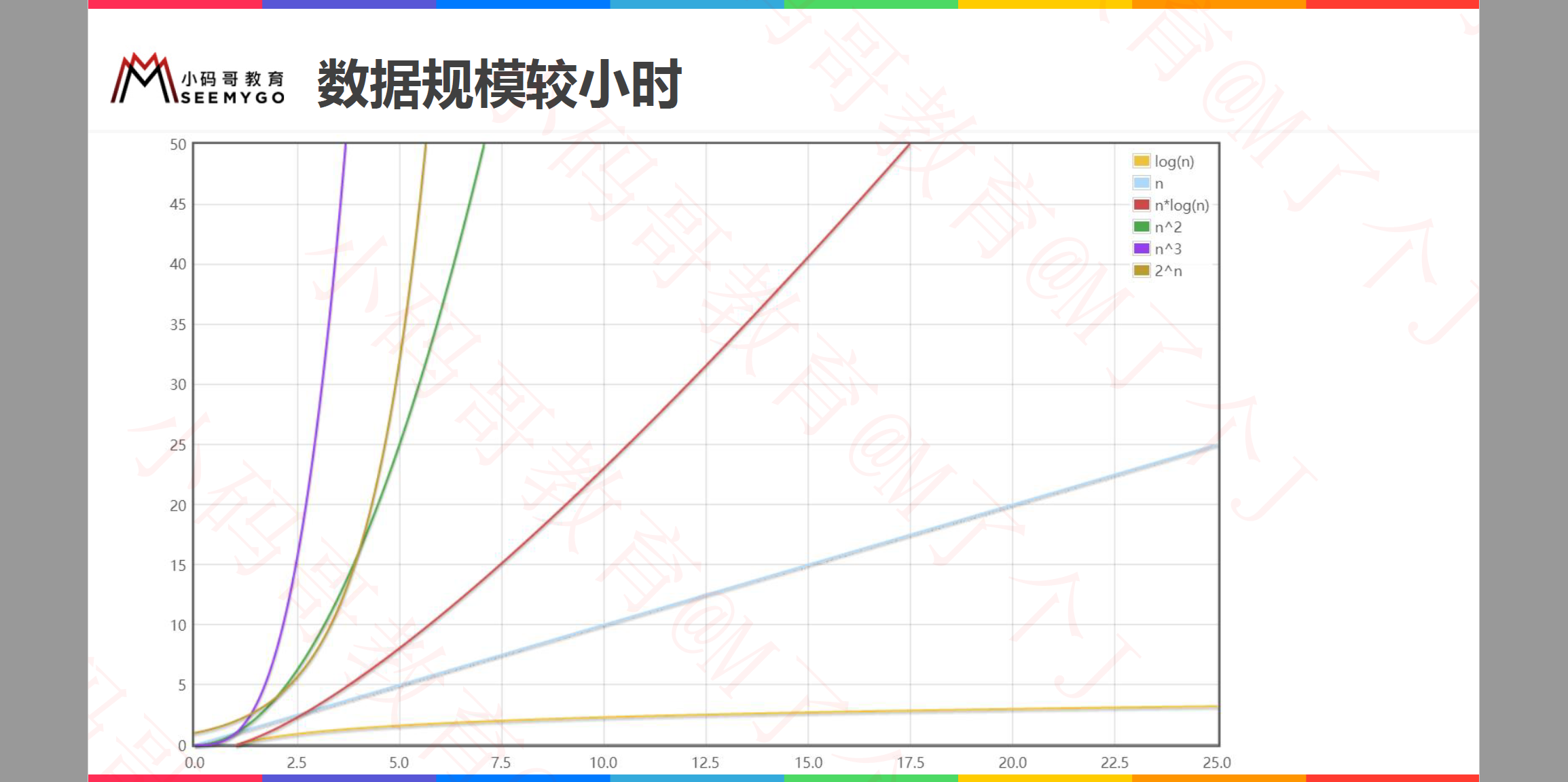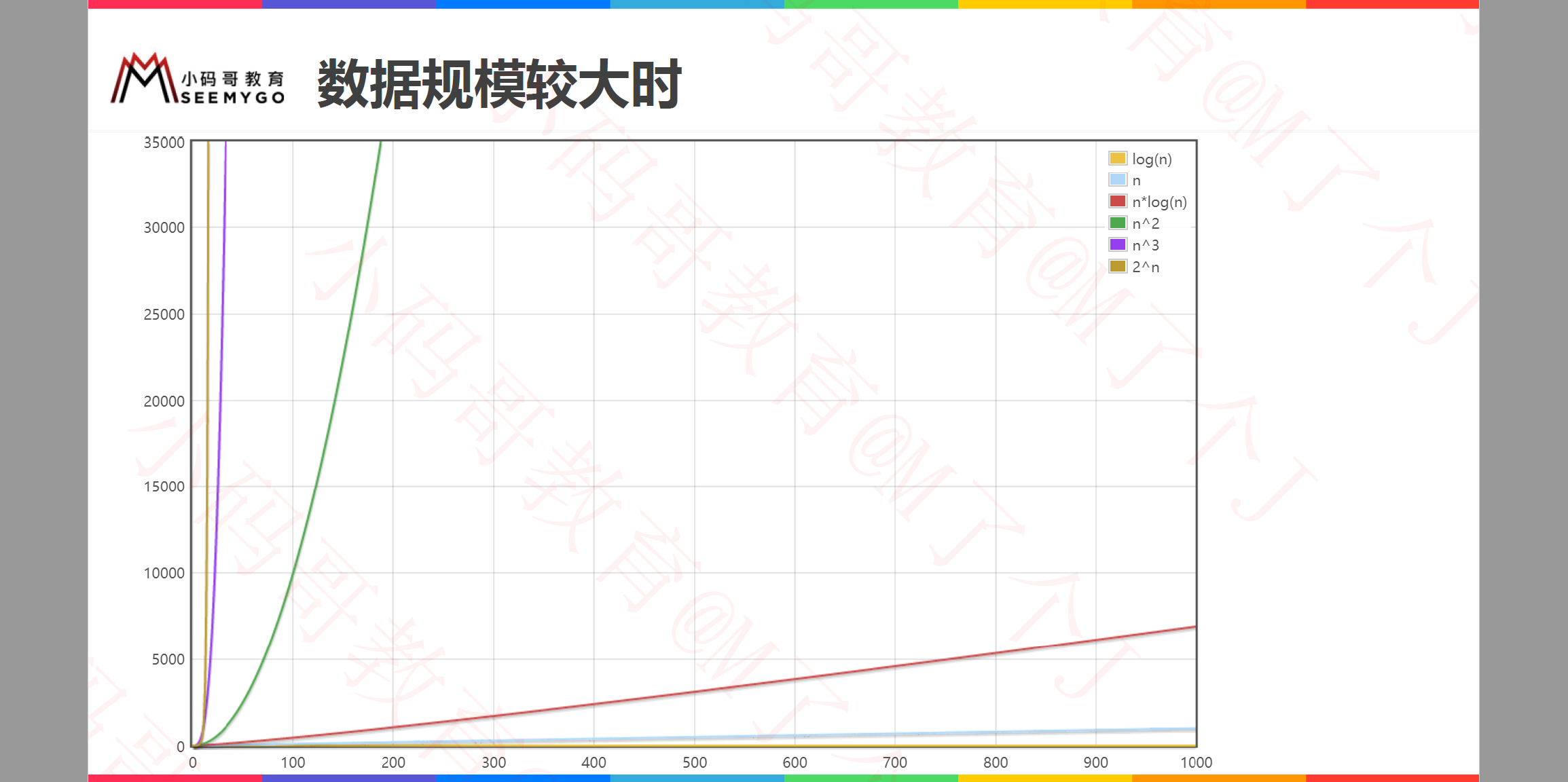# Leetcode

https://leetcode-cn.com/

https://leetcode.com/

https://leetcode-cn.com/problems/fibonacci-number/

# 斐波那契数列复杂度分析

https://leetcode-cn.com/problems/fibonacci-number/

/* 0 1 2 3 4 5
* 0 1 1 2 3 5 8 13 ....
*/

// O(2^n)
public static int fib1(int n) {
if (n <= 1) return n;
return fib1(n - 1) + fib1(n - 2);
}

// O(n)
public static int fib2(int n) {
if (n <= 1) return n;

int first = 0;
int second = 1;
for (int i = 0; i < n - 1; i++) {
int sum = first + second;
first = second;
second = sum;
}
return second;
}

public static int fib3(int n) {
if (n <= 1) return n;

int first = 0;
int second = 1;
while (n-- > 1) {
second += first;
first = second - first;
}
return second;
}

public static int fib4(int n) {
double c = Math.sqrt(5);
return (int) ((Math.pow((1 + c) / 2, n) - Math.pow((1 - c) / 2, n)) / c);
}


## 斐波那契数列-递归

// O(2^n)
public static int fib1(int n) {
if (n <= 1) return n;
return fib1(n - 1) + fib1(n - 2);
}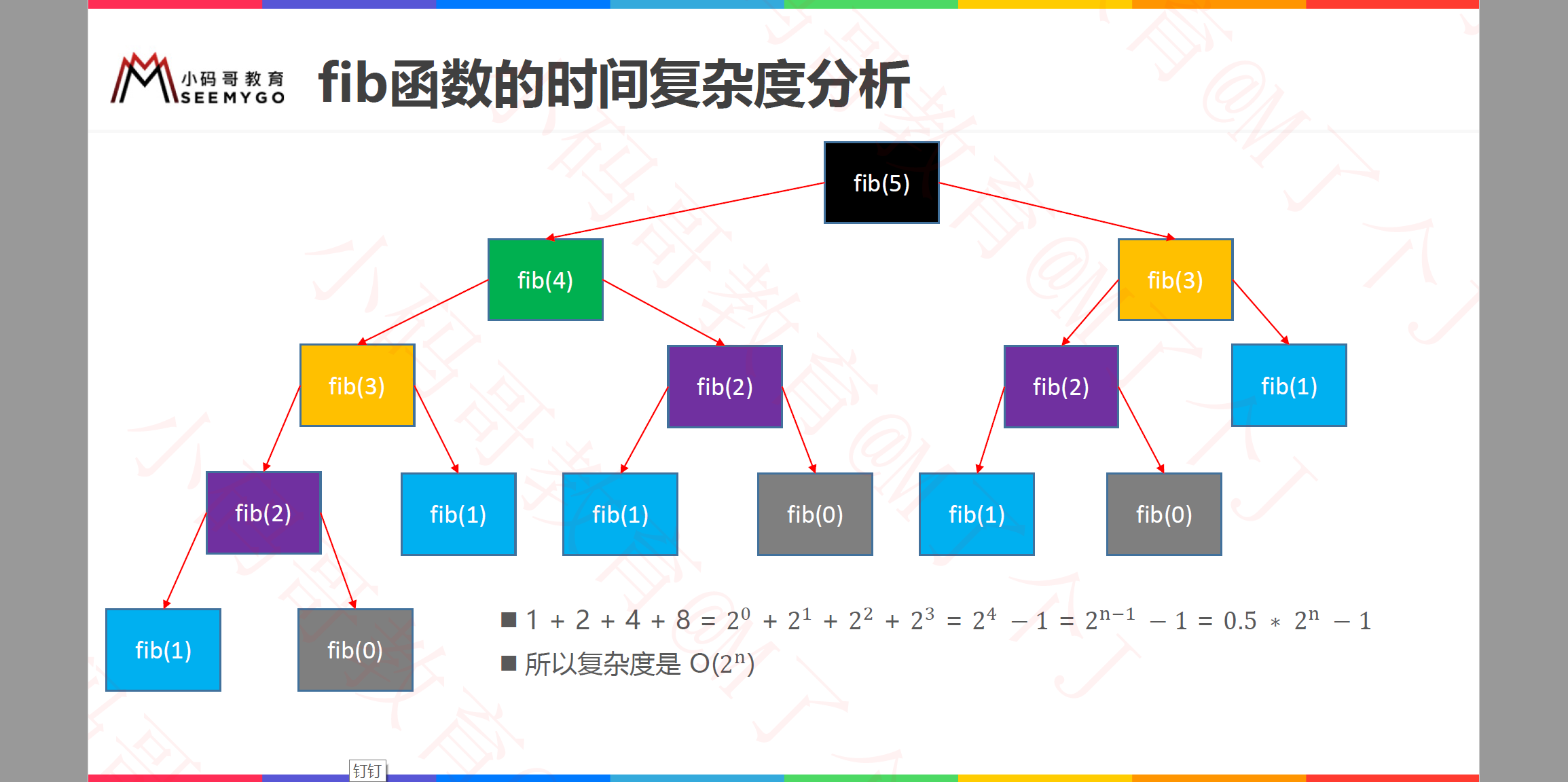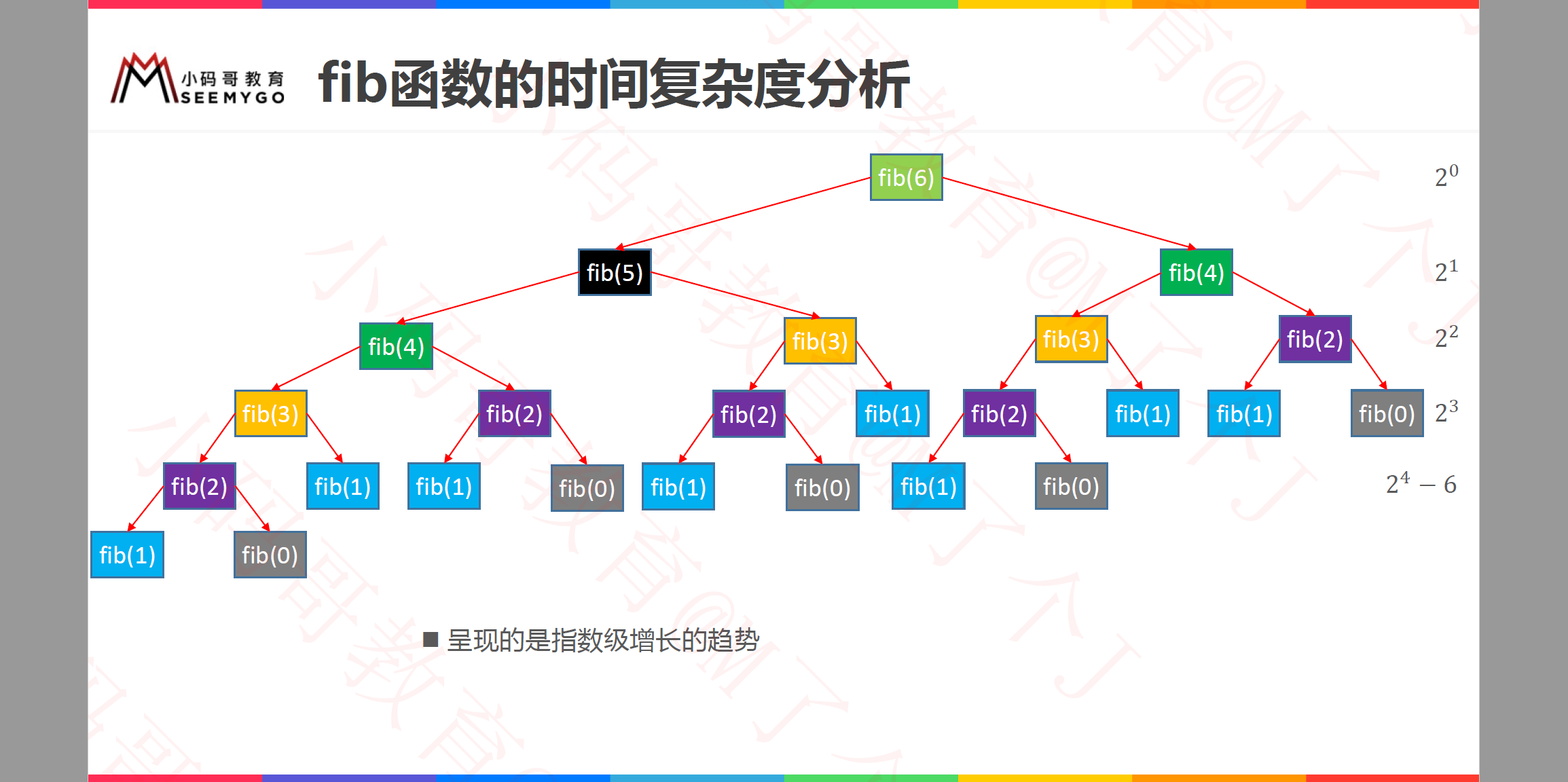## 斐波那契数列-循环

// O(n)
public static int fib2(int n) {
if (n <= 1) return n;

int first = 0;
int second = 1;
for (int i = 0; i < n - 1; i++) {
int sum = first + second;
first = second;
second = sum;
}
/*
// 也可以使用while循环
while (n-- > 1) {
second += first;
first = second - first;
}
*/
return second;
}



public static int fib3(int n){
if(n <= 1) return n;

int[] fib = new int[n+1];
fib = 0;
fib = 1;
for(int i = 2; i < fib.length; i++){
fib[i] = fib[i-1] + fib[i-2];
}
return fib[n];
}



## fib函数的时间复杂度分析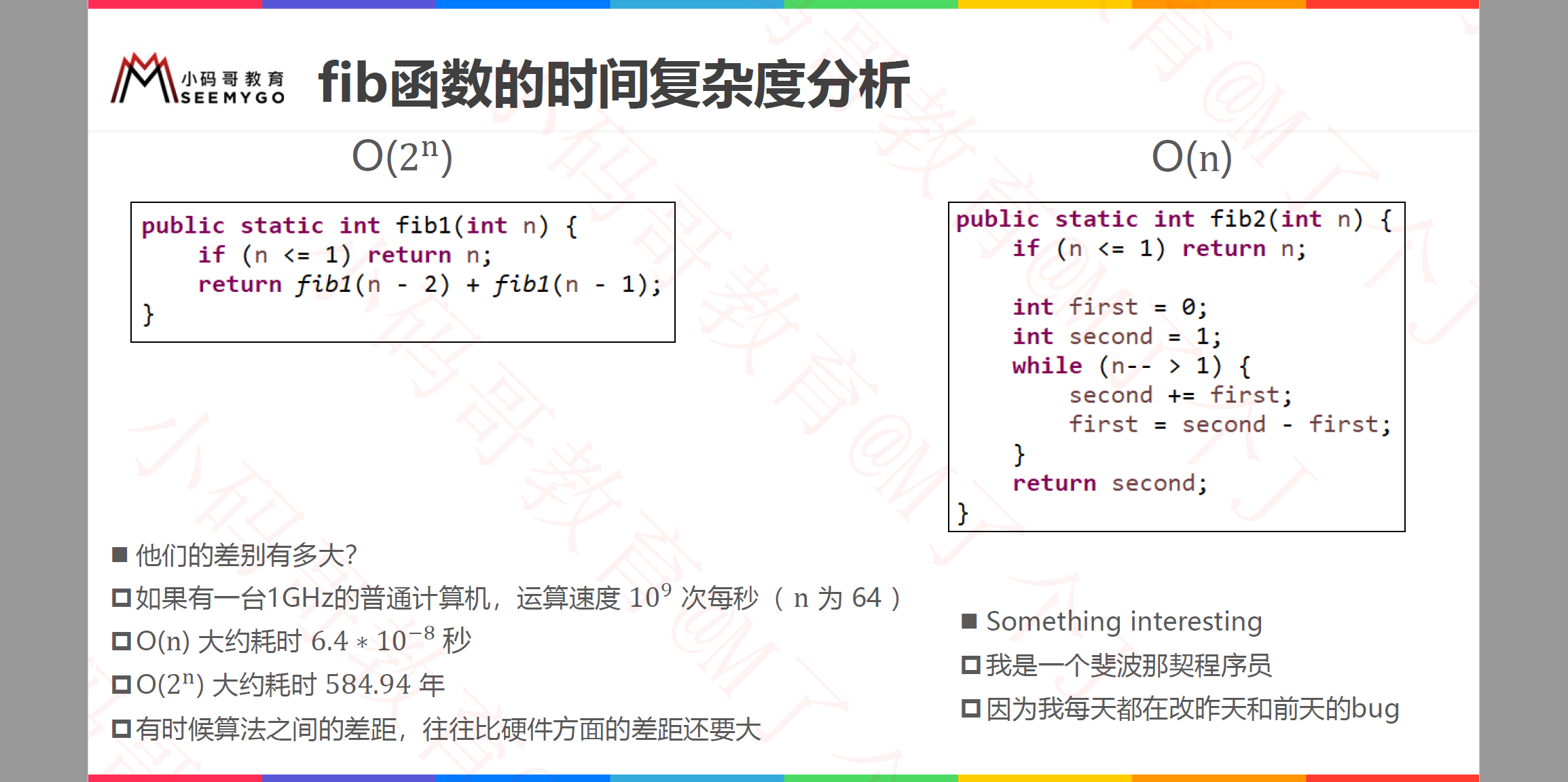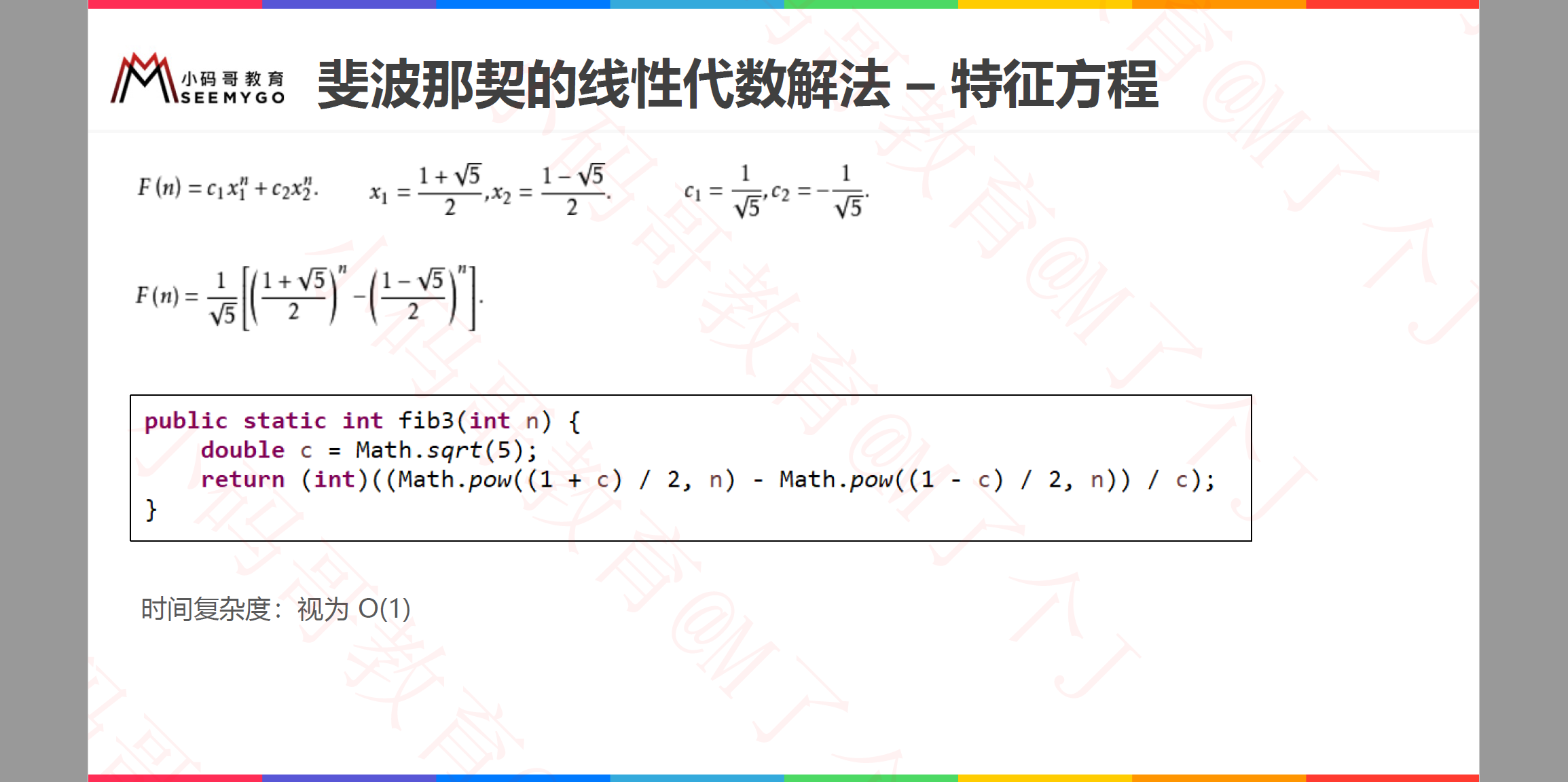# 多个数据规模的情况

public static void test(int n, int k){
for(int i = 0; i < n; i++){
System.out.println("test");
}
for (int i = 0; i < k; i++){
System.out.println("test");
}
}



# 算法的优化方向

• 用尽量少的存储空间
• 用尽量少的执行步骤（执行时间）
• 根据情况，可以
• 空间换时间
• 时间换空间

# 更多知识10-013万+

#### 斐波那契数列递归算法和非递归算法以及其时间复杂度分析

08-151182

#### 斐波那契数列两种算法及复杂度

09-074186

#### 斐波那契数列两种算法的时间复杂度

03-26691

#### 【恋上数据结构】复杂度知识以及LeetCode刷题指南

06-092037

#### 恋上数据结构与算法（精简版）

08-041万+

#### 斐波那契数列算法及时间复杂度分析

07-1229

#### 恋上数据结构与算法：B树(十六)

05-02913

#### LeetCode-70 Climbing Stairs(斐波那契数列)©️2020 CSDN 皮肤主题: 大白 设计师: CSDN官方博客点击重新获取扫码支付1.余额是钱包充值的虚拟货币，按照1:1的比例进行支付金额的抵扣。
2.余额无法直接购买下载，可以购买VIP、C币套餐、付费专栏及课程。余额充值